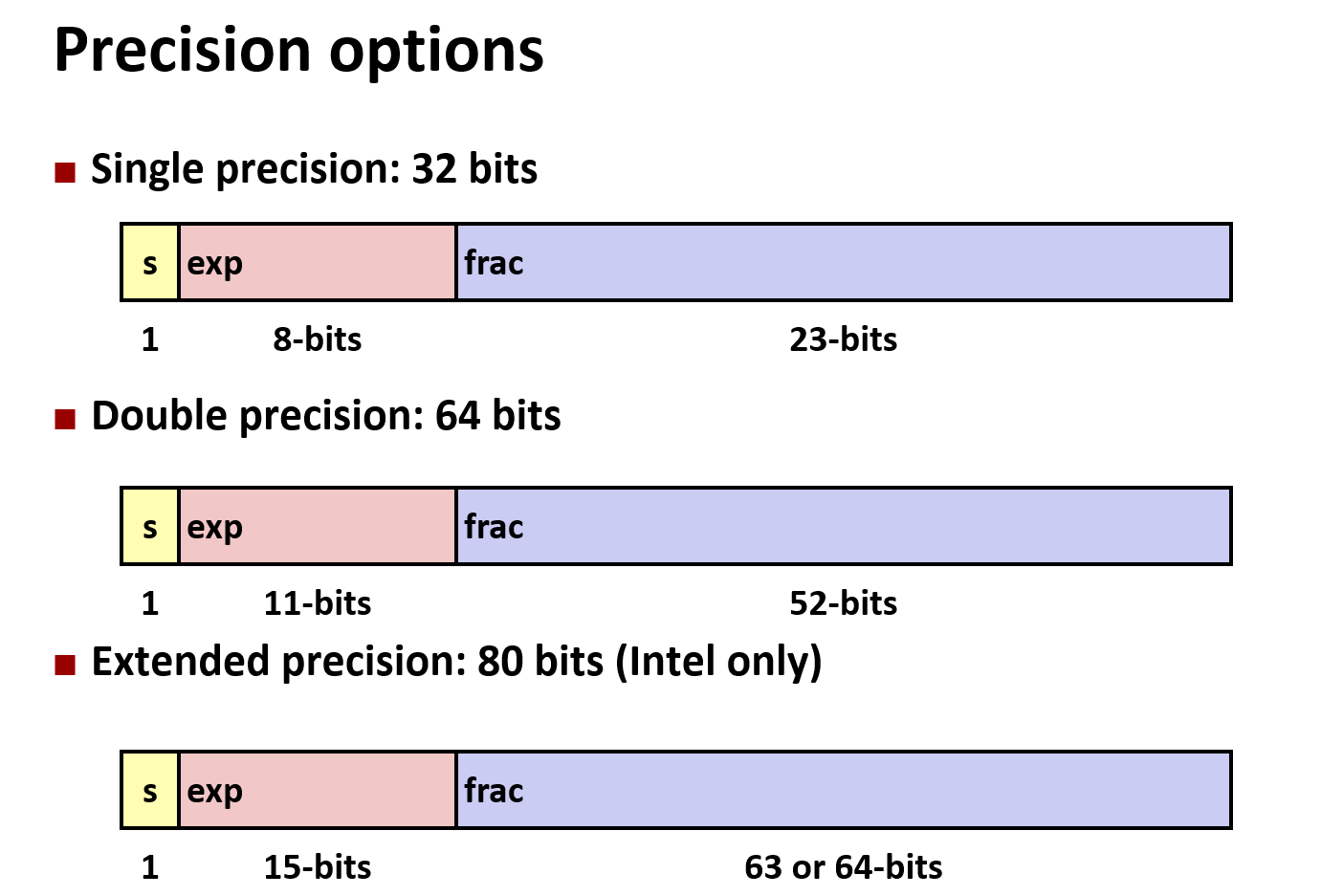## CSAPP 第二章笔记.md

tk_sky 18 2022-09-03

## 2. 信息表示法

### 2.1.1 十六进制

C语言中一般以0x0X开头表示十六进制值。

### 2.1.2 字数据大小

n位机器表示字长为n的机器。如32位和64位。

32位和64位程序在编译时编译器分配的字节数是不同的：

charunsigned char11
shortunsigned short22
intunsigned int44
longunsigned long48
int32_tuint32_t44
int64_tuint64_t88
float 44
double 88

### 2.1.3 寻址和字节顺序

• 小端法（大多数机器）：

• 大端法：

0x100 0x101 0x102

45 23 01 （其中45是最低有效字节）

0x100 0x101 0x102

01 23 45

### 2.1.6 布尔代数

• NOT 表示为 ~ 否

• AND 表示为 & 与

• OR 表示为 | 或

• EXCLUSIVE-OR 表示为 ^ 异或

### 2.1.9 位移运算

 >>4（逻辑右移）-> 

 >>4（算数右移）->

 >>4（逻辑右移）->

 >>4（算数右移）->

### 2.2.2 无符号数的编码

$$B2U_\omega(\vec x) = \sum\limits_{\omega-1}x_i2i$$

$$B2U_\omega$$ 是反身的，即 $$B2U_\omega$$ = $$U2B_\omega$$ ，因为二进制位及其表示的数是一一对应的。

### 2.2.3 补码编码

$$B2T_\omega(\vec x) = -x_{\omega-1}2{\omega-1}+ \sum\limits_{\omega-1}x_i2^i$$

$$B2T_4() = -02^3 + 122 +0*21 + 1*2^0 = 0+4+0+1 = 5$$

$$B2T_4() = -12^3 + 022 +1*21 + 1*2^0 = -8+0+2+1 = -5$$

• 原码

1：[0000 0001] -1：[1000 0001]

[+1] = 原 = 反 = 补

[-1] = 原 = 反 = 补

• 反码

[+1] = 原 = 反

[-1] = 原 = 反

### 2.2.4 有符号数与无符号数的转换

• 补码->无符号数

$$T2U_{\omega}(x)=x+2^{\omega} \space\space(x<0)$$

• 无符号数->补码

$$U2T_{\omega}(x)=x-2^{\omega} \space\space(x>TMax_{\omega})$$

c语言里面TMin不能写成-2147483648的原因_zerods-seu的博客-CSDN博客

### 2.2.6 扩展一个数的位表示

1. 无符号数的扩展：零扩展（扩展位全部填零）

2. 补码数的扩展：

$$B2T_{\omega+k}([x_{\omega-1},...,x_{\omega-1},x_{\omega-1},x_{\omega-2},...,x_0]) = B2T_\omega([x_{\omega-1},x_{\omega-2},...,x_0])$$

 = 8+3-16 = -5

 = 16+8+3-32 = -5

### 2.2.7 截断数字

1. 截断无符号数：

$$B2U_k[x_,x_,...,x_0]= B2U_\omega([x_{\omega-1},x_{\omega-2},...,x_0])\space mod\space 2^k$$

1. 截断补码数：

$$B2T_k[x_,x_,...,x_0]= U2T_k( B2U_\omega([x_{\omega-1},x_{\omega-2},...,x_0])\space mod\space 2^k )$$

0000000000000
0010010222222
10010019191-71
1011011B3113-53
1111111F7157-1-1

### 2.3.2 补码加法

$$x+y=x+y-2^\omega$$（正溢出）

$$x+y=x+y+2^\omega$$（负溢出）

### 2.3.4 无符号和补码乘法

$$x*y = (x ×y)mod\space2^\omega$$

### 2.3.6 乘/除以常数

$$x*2^k = x<<2$$

$$x/2^k = x>>2$$

### 2.4.2 IEEE浮点表示

IEEE浮点标准用类似科学计数法的方式来表示小数。

IEEE浮点标准表示法定义：

$$V = (-1)s\times M \times 2E$$

S：符号位 0/1

M：尾数 表示一个整数，相当于上述的原数

E：阶码 表示一个整数，作用是为尾数加权 类似于上述2的指数

s、exp、frac这里指符号数、阶码、尾数在浮点数数据类型中的位模式，不是它们的值IEEE定义了三种情况的值：

1. 规格化的值

e指浮点数exp位的值，bias是偏置值，因为E需要能够表示负数，所以减去偏置值使得阶码的值的范围能够与0对称。

bias的值为$$2^-1$$ （单精度为127，双精度为1023）

f 指的是frac区域的位表示的整数值。在解析时给他+1，目的是为了和非规格化数平滑衔接。

1. 非规格化的值

1. 特殊值

• 小数域（frac）全为0时，表示无穷，正还是负无穷由符号位决定。

• 小数域为非0时，表示NAN，即Not A Number，用来表示结果不能是实数或者无穷的情况，如计算$$\sqrt{-1}$$、无穷大-无穷大时就会返回NAN。

![aaaaa](CSAPP 第二章笔记.assets/aaaaa.png)

![img](CSAPP 第二章笔记.assets/MCVX`I%HS@MY{BOJ9D_PI5.png)

### 2.4.4 舍入

• 对于正好处于两个可表示小数值中间的数，IEEE标准采用向偶数舍入的方法，即向舍入后最末位为偶数的方向进行舍入。

![bbb](CSAPP 第二章笔记.assets/bbb.png)

• 对于其他数，向更靠近的那个可表示的浮点数舍入。